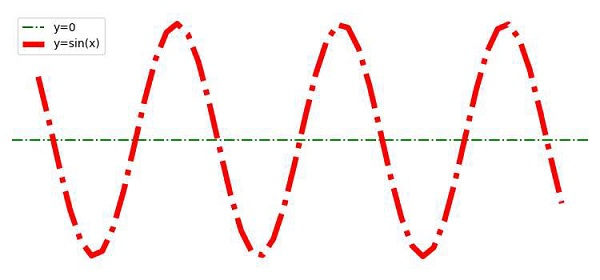# How to hide axes and gridlines in Matplotlib?

To hide axes (X and Y) and gridlines, we can take the following steps −

• Create x and y points using numpy.

• Plot a horizontal line (y=0) for X-Axis, using the plot() method with linestyle, labels.

• Plot x and y points using the plot() method with linestyle, labels.

• To hide the grid, use plt.grid(False).

• To hide the axes, use plt.axis('off')

• To activate the labels' legend, use the legend() method.

• To display the figure, use the show() method.

## Example

import numpy as np
from matplotlib import pyplot as plt
plt.rcParams["figure.figsize"] = [7.50, 3.50]
plt.rcParams["figure.autolayout"] = True
x = np.linspace(-10, 10, 50)
y = np.sin(x)
plt.axhline(y=0, c="green", linestyle="dashdot", label="y=0")
plt.plot(x, y, c="red", lw=5, linestyle="dashdot", label="y=sin(x)")
plt.grid(False)
plt.axis('off')
plt.legend()
plt.show()

## Output